RD Sharma Class 12 Solutions Chapter 15 Mean Value Theorems Ex 15.2 are part of RD Sharma Class 12 Solutions. Here we have given RD Sharma Class 12 Solutions Chapter 15 Mean Value Theorems Ex 15.2.

Here you can get free RD Sharma Solutions for Class 12 Maths of Chapter 15 Mean Value Theorems Exercise 15.2. All RD Sharma Book Solutions are given here exercise wise for Mean Value Theorems. RD Sharma Solutions are helpful in the preparation of several school level, graduate and undergraduate level competitive exams. Practicing questions from RD Sharma Mathematics Solutions for Class 12 Chapter 15 Mean Value Theorems is proven to enhance your math skills.

 Class: 12th Class Chapter: Chapter 15 Name: Mean Value Theorems Exercise: Exercise 15.2

## RD Sharma Class 12 Solutions Chapter 15 Mean Value Theorems Ex 15.2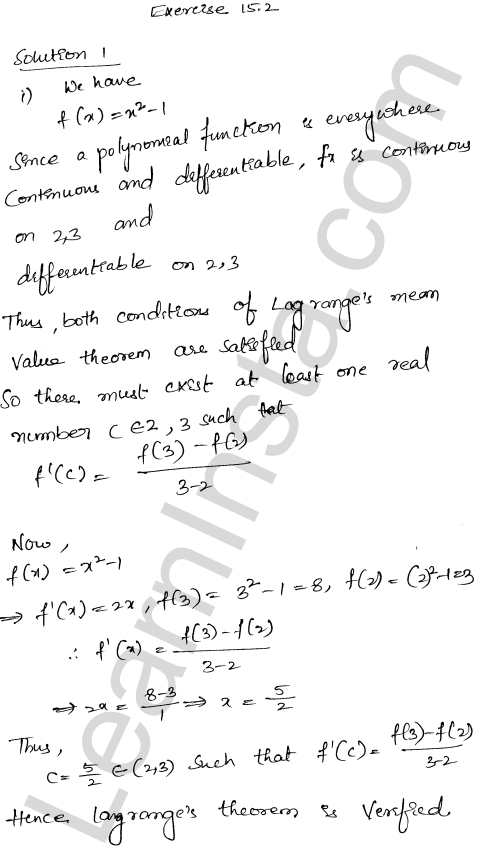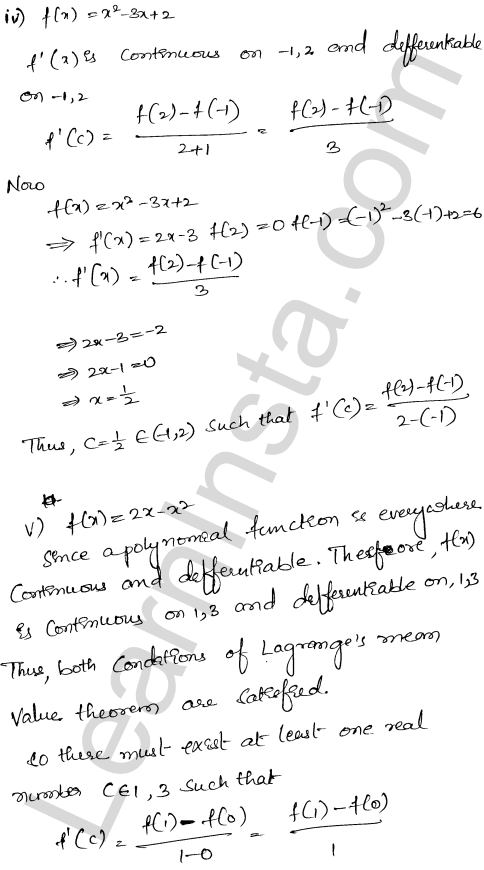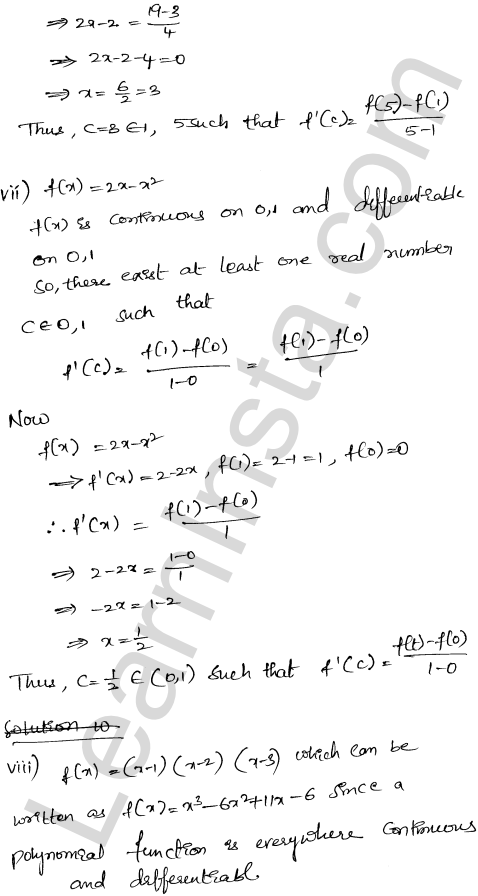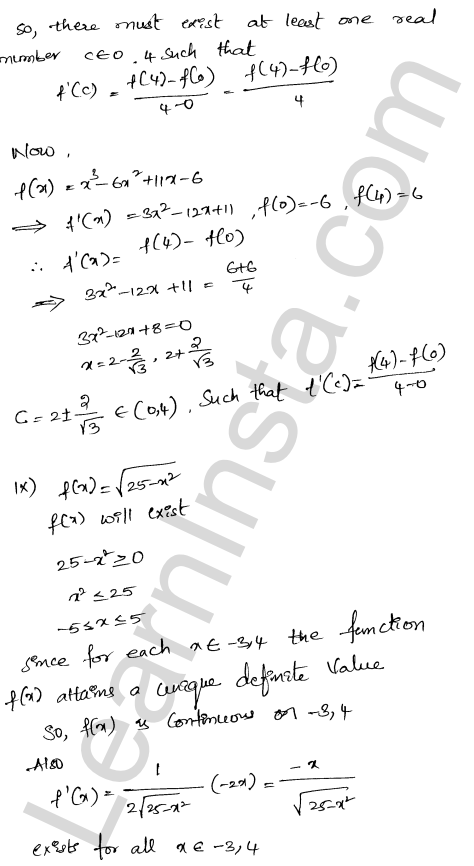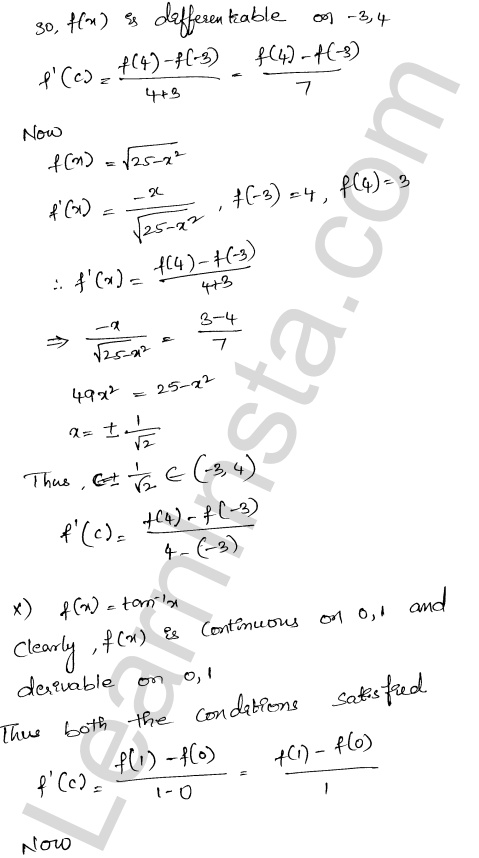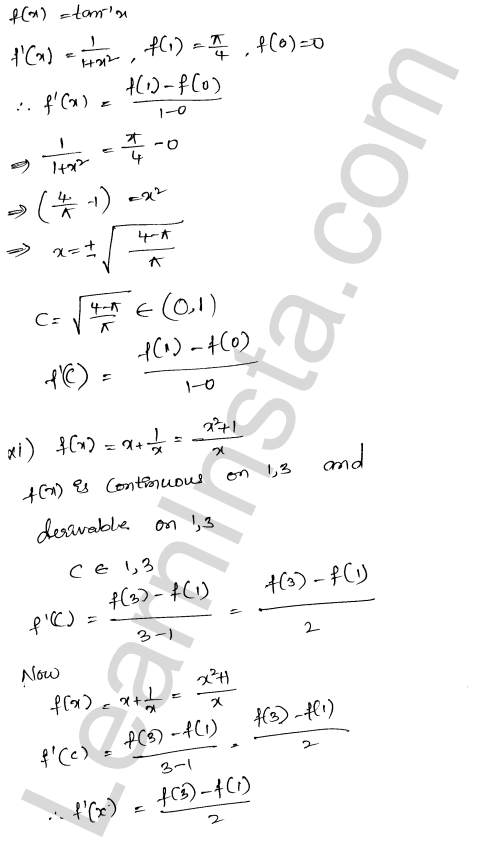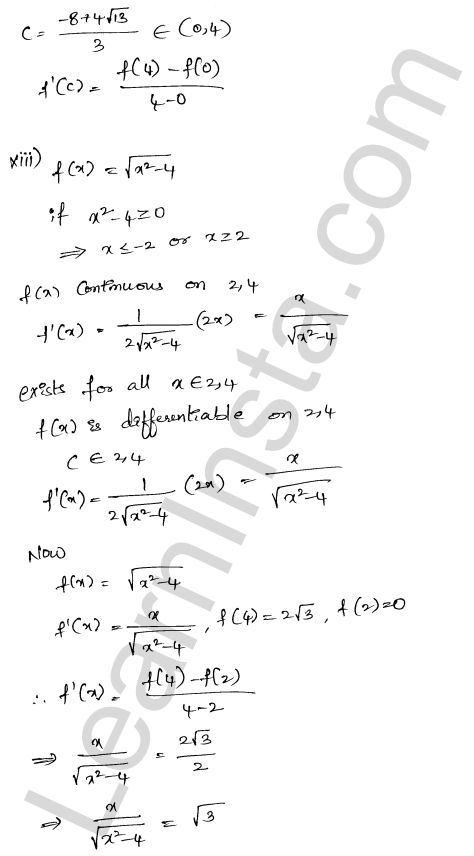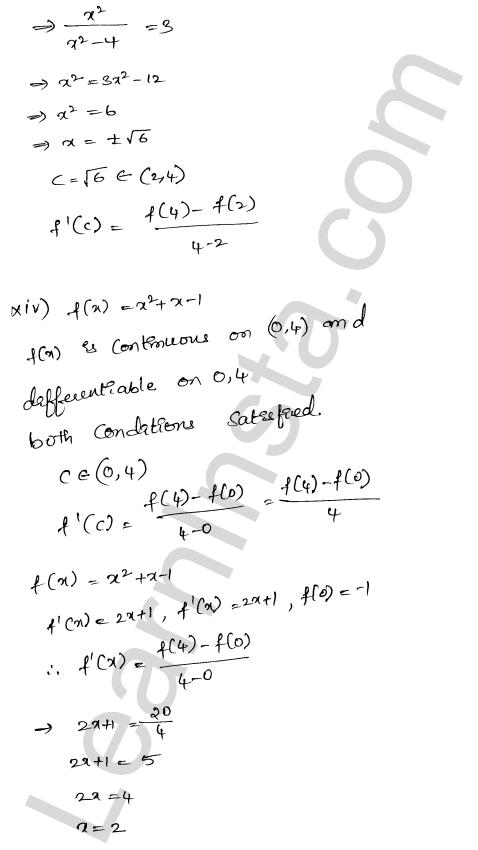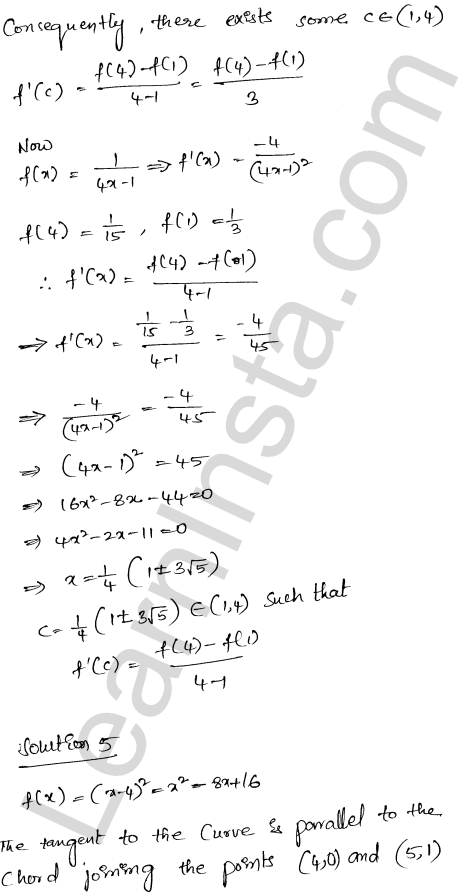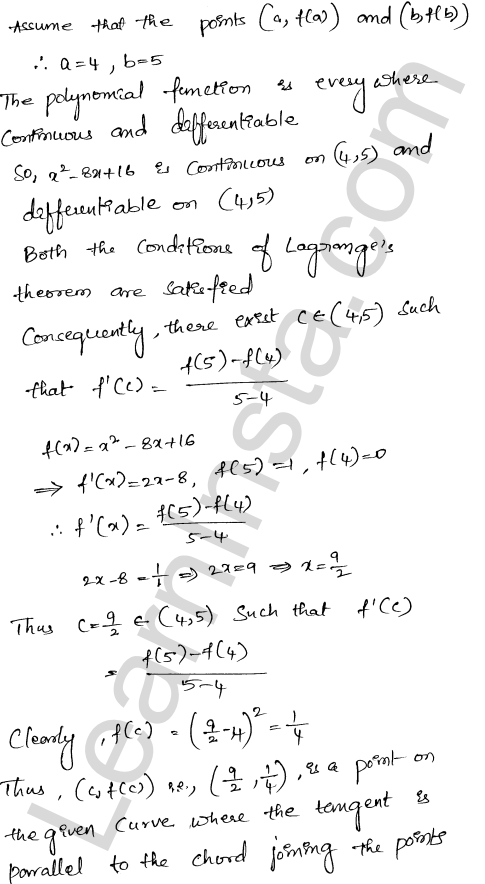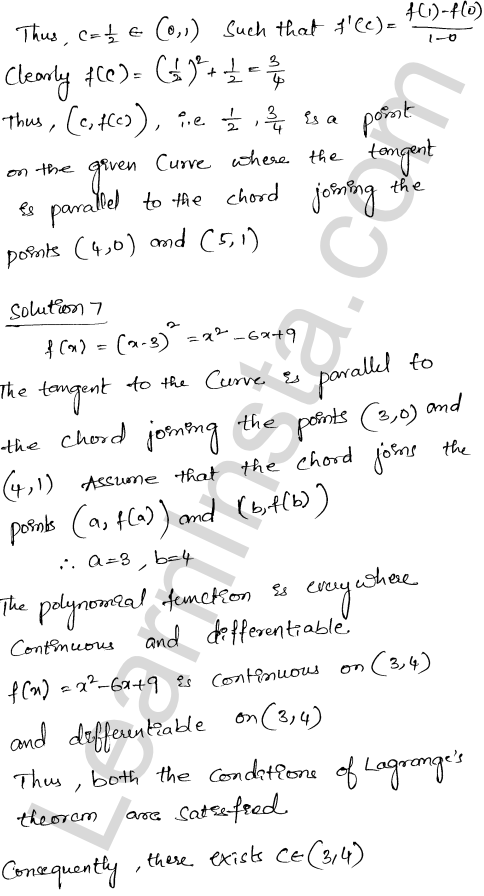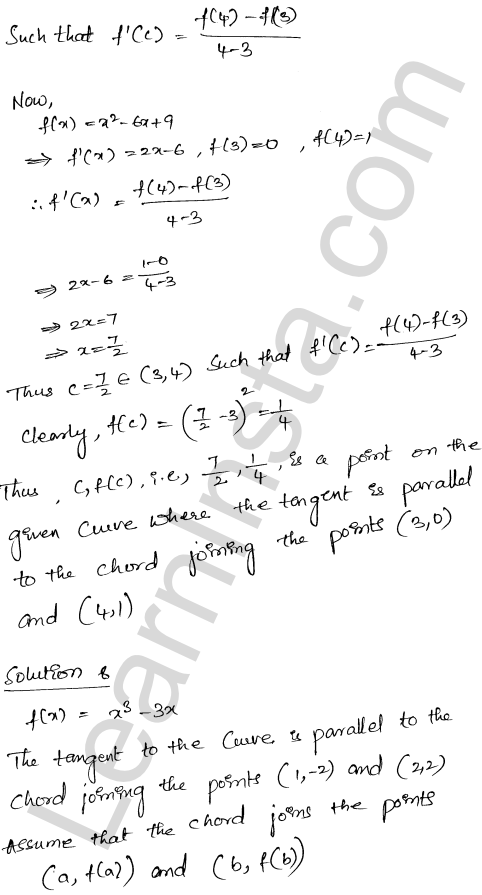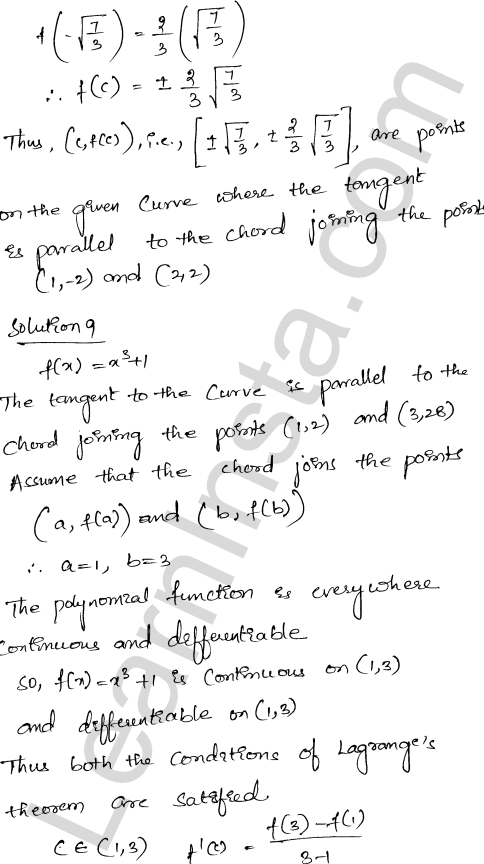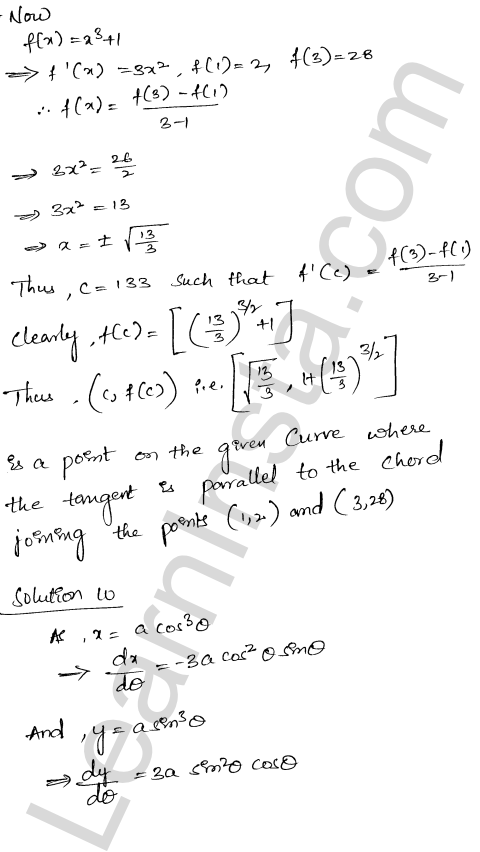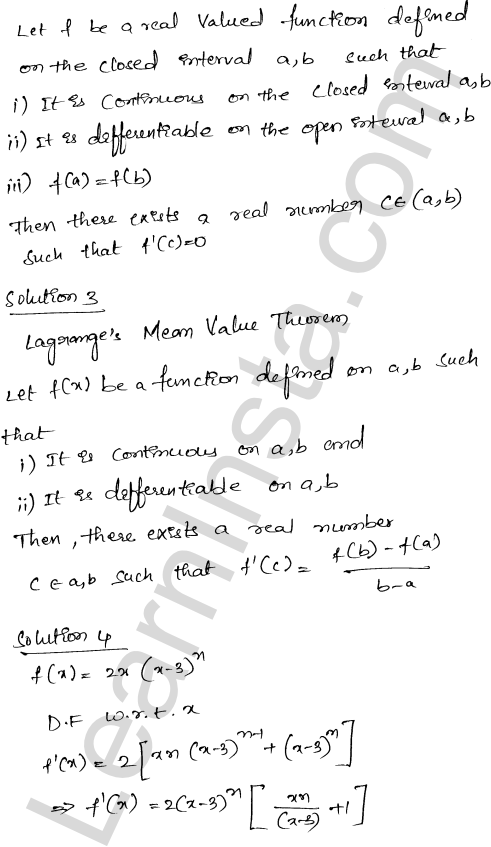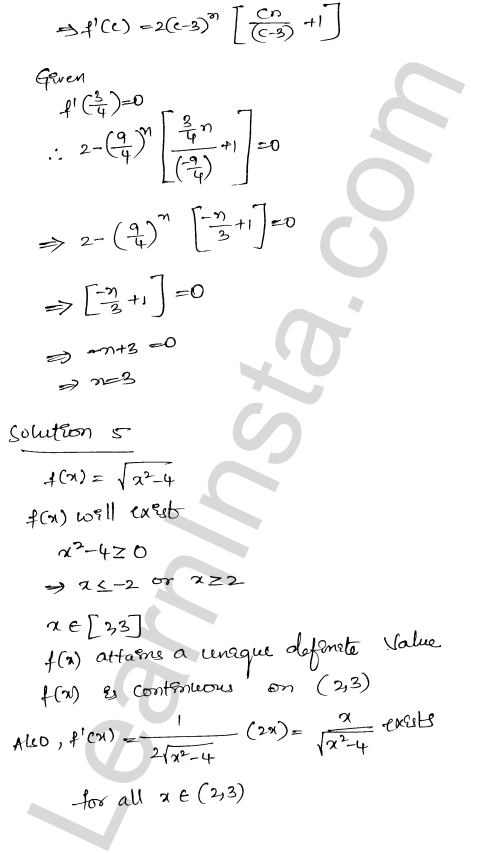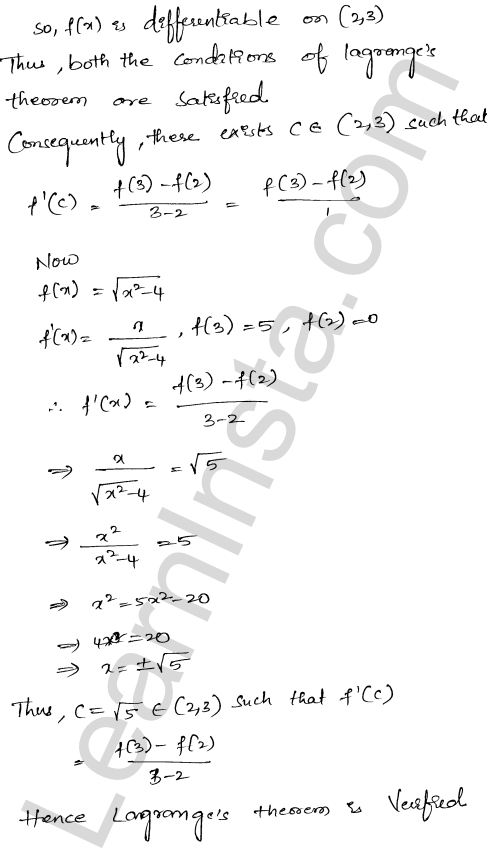We hope the RD Sharma Class 12 Solutions Chapter 15 Mean Value Theorems Ex 15.2, help you. If you have any query regarding RD Sharma Class 12 Solutions Chapter 15 Mean Value Theorems Ex 15.2, drop a comment below and we will get back to you at the earliest.